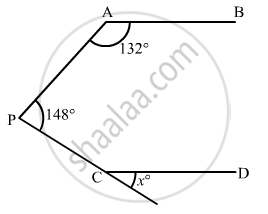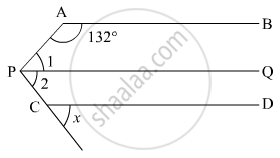# In the Given Figure, If Ab || Cd, Then X = - Mathematics

MCQ

In the given figure, if AB || CD, then x =• 100°

• 105°

• 110°

• 115°

#### Solution

The given figure is as follows:It is given that AB || CD.

Let us draw a line PQ parallel to AB and CD.

It is given that,

∠1 +∠2 = 148° (i)

Since, AB || PQ Thus,∠APQangle and ∠1 are consecutive interior angles.

Therefore,

∠1 + 132° = 180°

∠1 = 180° - 132°

∠1 = 48°          .. (ii)

Similarly, CD || PQ. Thus, angle and ∠2 are corresponding angles.

Therefore,

∠2 = x        (iii)

On substituting (ii) and (iii) in (i):

48° + x = 148°

x = 148° - 48°

x = 100°

Is there an error in this question or solution?
Chapter 10: Lines and Angles - Exercise 10.6 [Page 55]

#### APPEARS IN

RD Sharma Mathematics for Class 9
Chapter 10 Lines and Angles
Exercise 10.6 | Q 21 | Page 55

Share